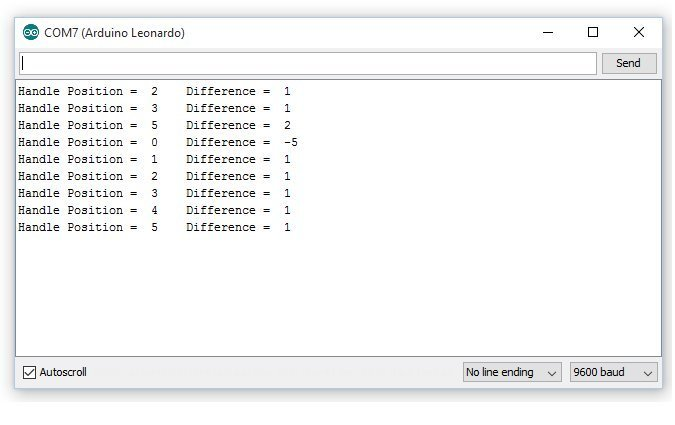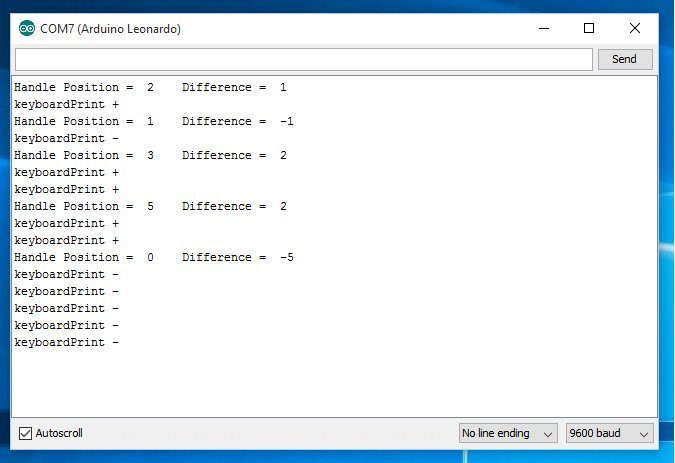# Potentiometer keyboardPrint challange

Hi all,

I want to keyboardPrint (HID) a letter based upon voltage or resistance change in Analog input A0. Does anyone know how to achieve this without the code ending in a repeated keyboardPrint? I just want to send a letter once after a change and wait for another change before sending a letter again.

I want:

When the lever goes UP, keyboardPrint “a”
When the lever goes DOWN, keyboardPrint “b”

hej,

have you tried a simple if else condition like:

``````int sensorPin = A0;    // select the input pin for the potentiometer
int sensorValue = 0;  // variable to store the value coming from the sensor
int storeValue = 0;

void setup() {
}

void loop() {
// read the value from the sensor:

if (storeValue == sensorValue) {

storeValue = sensorValue;
}
else {
}
}``````

That looks good! But this code will run in a loop pressing a zillion “a”`s.

I got this code, its almost perfect, it just needs little extra code but I don`t know how to to build it.

[code]

unsigned long startTime, endTime = 1000;
byte handlePos, oldHandlePos, currentPos;
int8_t diff;
int val;
bool posSent = true;

void setup()
{
Serial.begin(9600);
val = getAnalog();
Serial.print("\nCurrent handle position = “);
Serial.print(handlePos);
Serial.println(”\n\n");
currentPos = oldHandlePos = handlePos;
}

void loop()
{
val = getAnalog();
if(handlePos != currentPos)
{
startTime = millis();
currentPos = handlePos;
posSent = false;
diff = 0;
}
if(!posSent && millis() - startTime > endTime)
{
Serial.print(“Handle Position = “);
Serial.print(handlePos);
Serial.print(”\t”);
diff = handlePos - oldHandlePos;
Serial.print("Difference = ");
Serial.println(diff);
oldHandlePos = handlePos;
posSent = true;
}
}

int getAnalog()
{
if(val < 187) handlePos = 0;
else if(val < 350) handlePos = 1;
else if(val < 518) handlePos = 2;
else if(val < 679) handlePos = 3;
else if(val < 861) handlePos = 4;
else handlePos = 5;
return val;
}
// by Jeroen van der Velden
// Credits to Arduino Forum member “OUTSIDER”[/code]I guess I need something like this;

IF handlepos is = 1 and previous handlepos = 4 THEN keyboardPrint("-") three times)
IF handlepos is = 5 and previous handlepos = 0 THEN keyboardPrint("+") five times)

Code found!

The code below will now print - and + depending on direction movement of the Retarder Lever (up or down) and if it goes up or down multiple positions at once it will calculate and send the appropriate - and + characters to your Simulator.

Topic may close now…

[code]unsigned long startTime, endTime = 1000;
byte handlePos, oldHandlePos, currentPos;
int8_t diff;
int val;
bool posSent = true;

void setup()
{
Serial.begin(9600);
val = getAnalog();
Serial.print("\nCurrent handle position = “);
Serial.print(handlePos);
Serial.println(”\n\n");
currentPos = oldHandlePos = handlePos;
}

void loop()
{
val = getAnalog();
if(handlePos != currentPos)
{
startTime = millis();
currentPos = handlePos;
posSent = false;
diff = 0;
}
if(!posSent && millis() - startTime > endTime)
{
Serial.print(“Handle Position = “);
Serial.print(handlePos);
Serial.print(”\t”);
diff = handlePos - oldHandlePos;
Serial.print("Difference = ");
Serial.println(diff);

``````// if diff is negative number to positive number
if(diff < 0)
{
for (int i = 0; i < (diff * -1); i++)
{
Keyboard.print("-");
}
}

// if diff is positive number to positive number
if(diff > 0)
{
for (int i = 0; i < diff; i++)
{
Keyboard.print("+");
}
}

oldHandlePos = handlePos;
posSent = true;
}
``````

}

int getAnalog()
{
if(val < 187) handlePos = 0;
else if(val < 350) handlePos = 1;
else if(val < 518) handlePos = 2;
else if(val < 679) handlePos = 3;
else if(val < 861) handlePos = 4;
else handlePos = 5;
// Code by “Jeroen van der Velden”but anyway: closed